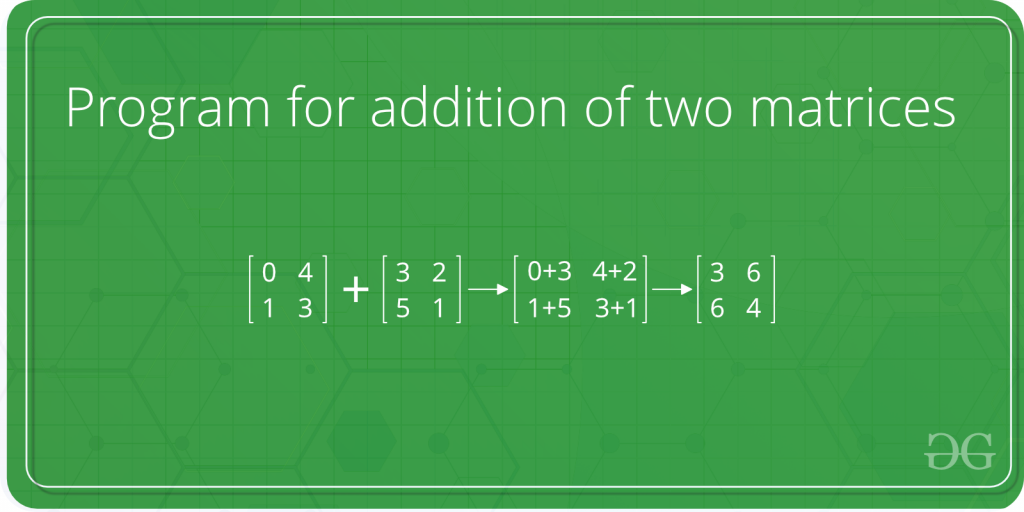Open in App
Not now

# C Program to Add Two Matrices

• Last Updated : 26 Oct, 2022The below program adds two square matrices of size 4*4, we can change N for different dimensions.

## C

 `// C program to implement ` `// the above approach ` `#include ` `#define N 4 ` ` `  `// This function adds A[][] and B[][],  ` `// and stores the result in C[][] ` `void` `add(``int` `A[][N], ``int` `B[][N],  ` `         ``int` `C[][N]) ` `{ ` `    ``int` `i, j; ` `    ``for` `(i = 0; i < N; i++) ` `        ``for` `(j = 0; j < N; j++) ` `            ``C[i][j] = A[i][j] + B[i][j]; ` `} ` ` `  `// Driver code ` `int` `main() ` `{ ` `    ``int` `A[N][N] = {{1, 1, 1, 1}, ` `                   ``{2, 2, 2, 2}, ` `                   ``{3, 3, 3, 3}, ` `                   ``{4, 4, 4, 4}}; ` ` `  `    ``int` `B[N][N] = {{1, 1, 1, 1}, ` `                   ``{2, 2, 2, 2}, ` `                   ``{3, 3, 3, 3}, ` `                   ``{4, 4, 4, 4}}; ` ` `  `    ``// To store result ` `    ``int` `C[N][N];  ` `    ``int` `i, j; ` `    ``add(A, B, C); ` ` `  `    ``printf``(``"Result matrix is "``); ` `    ``for` `(i = 0; i < N; i++) ` `    ``{ ` `        ``for` `(j = 0; j < N; j++) ` `           ``printf``(``"%d "``, C[i][j]); ` `        ``printf``(``""``); ` `    ``} ` ` `  `    ``return` `0; ` `} `

Output:

```Result matrix is
2 2 2 2
4 4 4 4
6 6 6 6
8 8 8 8```

The program can be extended for rectangular matrices. The following post can be useful for extending this program.
How to pass a 2D array as a parameter in C?
The time complexity of the above program is O(n2).

The auxiliary space of the above problem is O(n2)

My Personal Notes arrow_drop_up
Related Articles